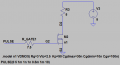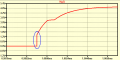The LTspice MOSFET switch artifact?

Jony130

Joined Feb 17, 2009
5,289
Here I have a simple MOSFET switching circuit.And the voltage at the gate looks like this:And is this "spike" (sharp edge) at the gate is a simulator artifact?
Also, form what I have noticed is If I remove the Rg resistance from the MOSFET model this "spike" disappears and I've got an exponential rise at the Vg as expected.
What do you think about it?

Attachments

• 2.2 KB Views: 8

crutschow

Joined Mar 14, 2008
28,545
And is this "spike" (sharp edge) at the gate is a simulator artifact?
No. A Spice simulator seldom has serious simulation "artifacts".
You will see the same effect in a real circuit.
It's the result of the "Miller effect" which magnifies the apparent value of the gate-drain capacitance by the gain of the circuit when the MOSFET starts to turn on .
This effect is not seen until after the MOSFET's Vgs(thres) voltage is reached, so that is the fast rise-time "spike" you are seeing
If you look at the collector voltage you will see that delay.
It's the charging of this Miller capacitance through the input resistor that slows the rise of the gate voltage above that point.
If I remove the Rg resistance from the MOSFET model this "spike" disappears
Of course, because there is now no voltage drop across Rg due to the input Miller effect charging current.

Jony130

Joined Feb 17, 2009
5,289
Of course, because there is now no voltage drop across Rg due to the input Miller effect charging current.
But why the "external" RG does not create such a spike? Only "internal" Rg creates this spike. Also, why setting Rg = 3m Ohms brings back the exponential rise (reducing the gate resistance increases the time constant), something is not right here?

crutschow

Joined Mar 14, 2008
28,545
But why the "external" RG does not create such a spike? Only "internal" Rg creates this spike.
Because you can's see the effect of the "internal" Rg
why setting Rg = 3m Ohms brings back the exponential rise (reducing the gate resistance increases the time constant), something is not right here?
What do you mean "increases the time constant"?
Do you mean a faster rise-time or a slower rise-time?

Jony130

Joined Feb 17, 2009
5,289
What do you mean "increases the time constant"?
Do you mean a faster rise-time or a slower rise-time?
I meant the slower rise-time when "setting" internal gate resistance Rg to 3mΩ in my model declaration.

The thing I cannot understand is how the Cgd capacitor can "create" the sharp edge at the gate when we also have a Cgs capacitor.
As if this "internal" Rg resistor was connected between Cgd and Cgs.

I already this jus that. But here you have again.

Attachments

• 1.1 KB Views: 10

crutschow

Joined Mar 14, 2008
28,545
I already this jus that. But here you have again.
Sorry, I missed that.Okay, I believe I see what is happening.

I was mistaken, you are seeing the result of the internal Rg resistor.

With Rg=3 ohms (top simulation) the initial "spike" is simply the voltage division between the series connection of the external R_GATE1, and Rg at the start of the gate capacitance charging.
After that, the MOSFET starts to turn on when the internal gate voltage (after Rg) reaches the MOSFET threshold voltage (at about 2.6us) and the Miller affect changes the slope of the input charging curve to almost horizontal, due to the added Miller capacitance.
Once, the MOSFET has fully turned on (at about 3.2us), there is no more Miller effect and the curve resumes its exponential curve.

With Rg=3m (bottom simulation) there's no significant initial voltage drop across Rg so you see the charging curve from the R_GATE1 resistance starting from 0V.

Make sense now?that's exactly it,,, the gate resistance develops voltage first. But it also slows down the gate charge time and the current spike.